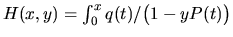Next: Discrete Geometry / Géométrie Up: Algebraic Geometry / Géométrie Previous: Evgenii Shustin - Asymptotically

## Yosef Yomdin - A tractable problem on the way (hopefully) to counting limit cycles of polynomial vector fields

 YOSEF YOMDIN, Weizmann Institute of Science, Rehovot, Israel A tractable problem on the way (hopefully) to counting limit cycles of polynomial vector fields

We show that a certain infinitesimal version of the problem of bounding the number of limit cycles of a polynomial vector field on the plane leads to an interesting question, which can be formulated very simply:

Let P(x) and q(x) be two polynomials. Consider the function.

1.  Find all x for which H(x,y) is identically zero in y.

2. For any fixed x estimate the number of (real) zeroes of H(x,y) in y.

These questions turn out to be closely related to several fields in Analysis and Algebra, in particular to the Generalized moments problem, to the algebra of polynomials under composition and to D-modules. We plan to present some of these relations, an answer to the questions 1 and 2 and to discuss some additional results and problems in the above direction.Next: Discrete Geometry / Géométrie Up: Algebraic Geometry / Géométrie Previous: Evgenii Shustin - Asymptotically